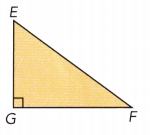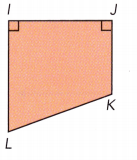# Math in Focus Grade 7 Chapter 6 Answer Key Angle Properties and Straight Lines

Practice the problems of Math in Focus Grade 7 Workbook Answer Key Chapter 6 Angle Properties and Straight Lines to score better marks in the exam.

## Math in Focus Grade 7 Course 2 B Chapter 6 Answer Key Angle Properties and Straight Lines

### Math in Focus Grade 7 Chapter 6 Quick Check Answer Key

Tell whether each angle is an acute, right, obtuse, or straight angle.

Question 1.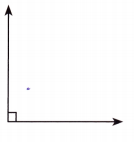Right angle triangle.

Explanation:
A right-angled triangle is a triangle with one of the angles as 90 degrees.

Question 2.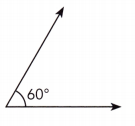Acute angle triangle.

Explanation:
An acute-angled triangle is a type of triangle in which all the three internal angles of the triangle measures less than 90°.

Question 3.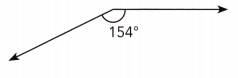90° < 154° < 180° We have
The given angle is obtuse because Its measure is greater than 90°, but Less than 180°.
obtuse

Question 4.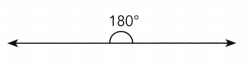straight angle.

Explanation:
The 180-degree angle is known as a straight angle. The sides of the angle are opposite to each other and they make a straight angle.

Question 5.
m∠w = 86°
Acute angle

Explanation: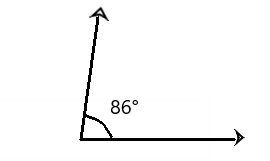Question 6.
m∠y = 90°
Right angle triangle.

Explanation: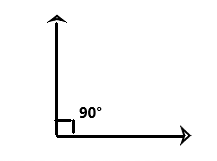Identify each pair of parallel line segments.

Question 7.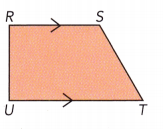RSUT

Explanation:
The lines will not meet each other at any point in the given figure. Hence parallel line will never intersect.

Question 8.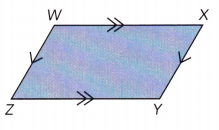WXZY

Explanation:
The lines that do not intersect or meet each other at any point in a plane. Parallel lines will never intersect.

Identify each pair of perpendicular line segments.

Question 9.
ABCD is a rectangle.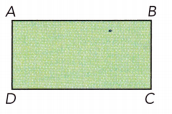There are four right angles, and 2 pairs of perpendicular lines.

Explanation:
A rectangle has lines that are perpendicular to two other lines and it also has four right angles.

Question 10.